# Create a Progress Bubble Chart in Tableau

### Objective:

Our objective is to build a progress chart for different KPIs.  In this example, we will be using: “Marketing Cost vs Budget”, “Operational Cost vs Budget” and “Headcount Cost vs Budget”. The Progress Chart will look like this: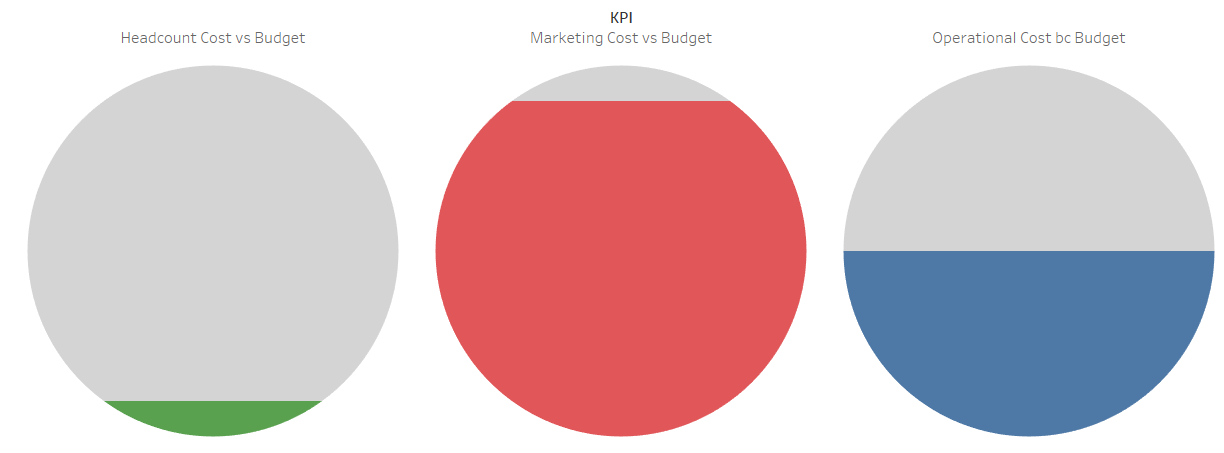### Pre-requisites:

To create this type of chart, we need to have data in the specific format. Take a look at the design of the data in this format.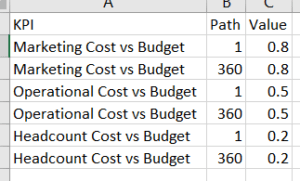### Data Summary:

Background Information:

As per the objective of building network chart, following fields are needed in a dataset:

1.  KPI: This field provides the KPI’s name like “Marketing Cost vs Budget”, “Operational Cost vs Budget” and “Headcount Cost vs Budget”
2. Path: For each KPI, we have defined two values of Path i.e. 1 and 360. This field is required to build this type of chart
3. Value: This is a numerical field showing a value of each KPI which is being shown in the Progress Chart later. The same value is being repeated corresponding to each path value

### Steps:

Following are the steps:

1. Connect to “Progress Chart.xlsx” data source. For reference, purpose data is attached along with this article
2. Go to Sheet1: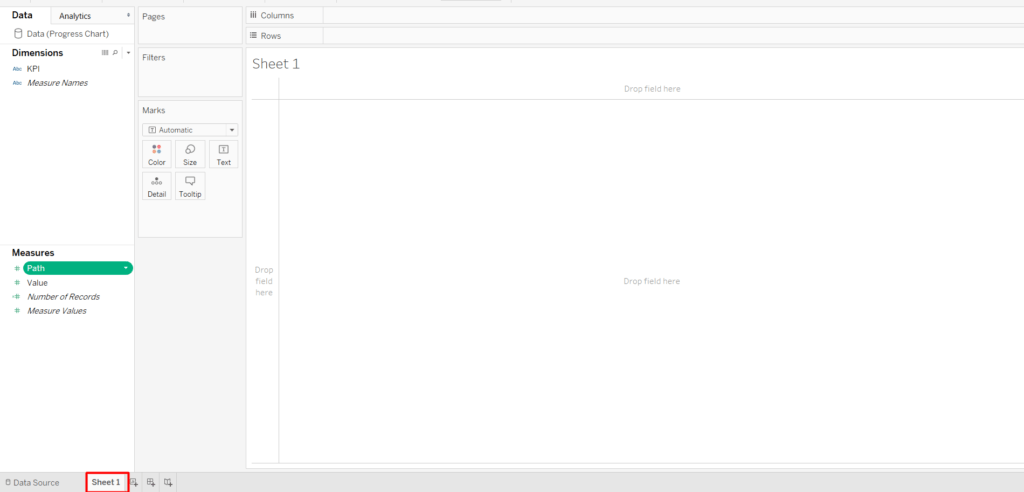3. Create “Bins” using the field “Path”
1. Go to the “Path” field in “Measures” and select “Create Bins…” option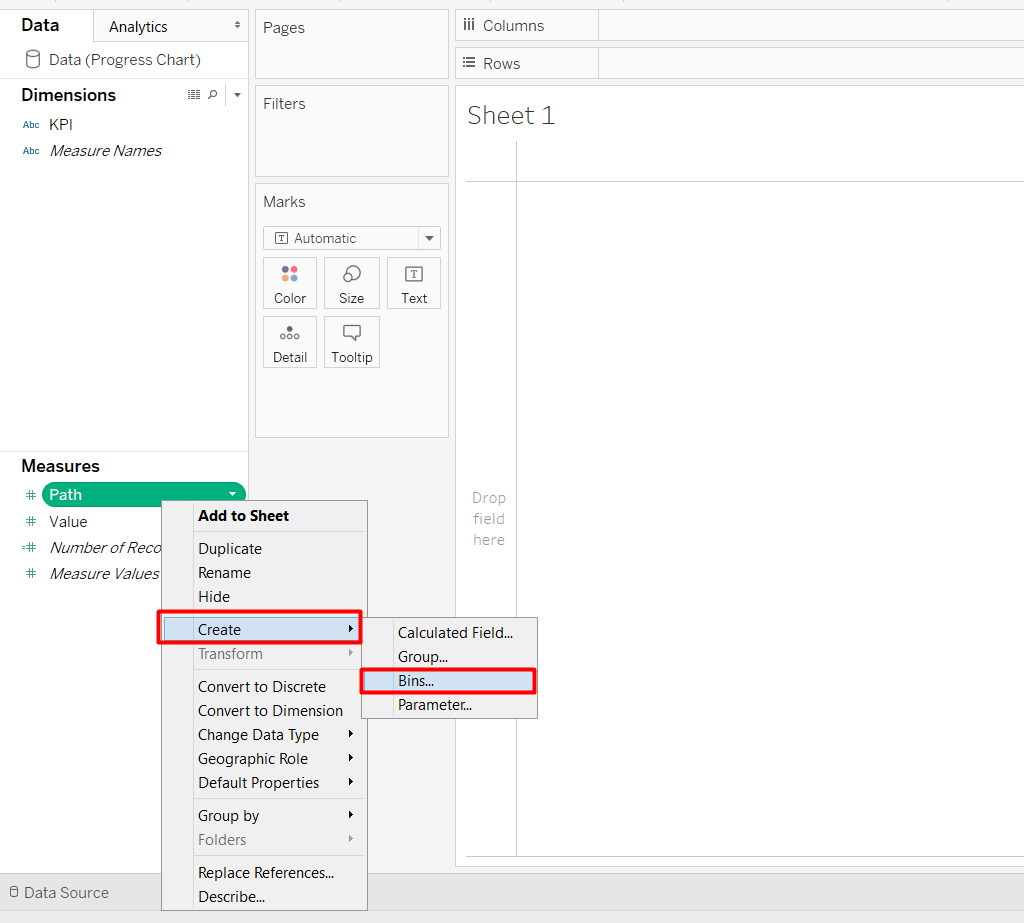2. Under “Size of bins:” option, enter value 1 and then click “OK”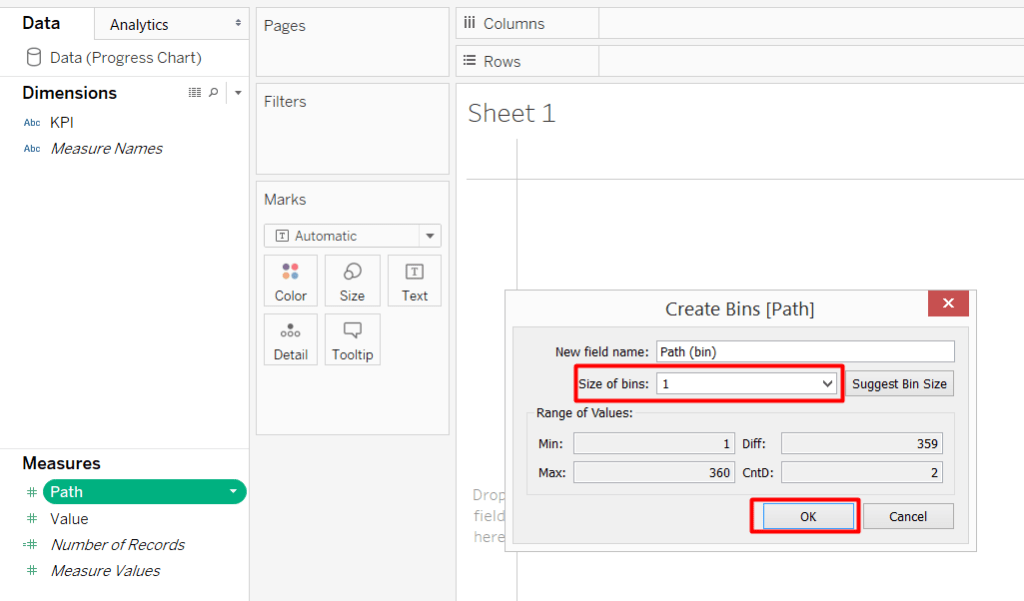4. Now, before we start to build the chart, there are some calculations that need to be created. Create the following mentioned calculations:
1. Index: calculation is- INDEX()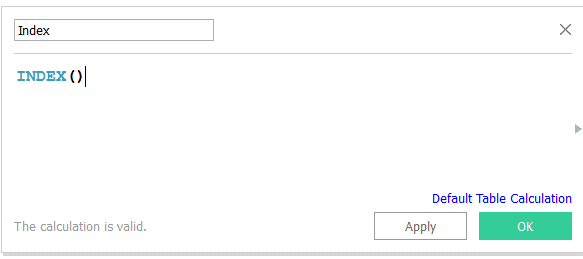2. PC_Value: This is used to show the value of each KPI. Calculation is- WINDOW_MAX(MAX([Value]))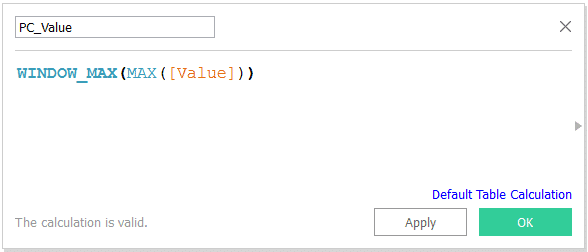3. PC_PI: This calculation is used to build the circles of Progress Chart. Calculation is- WINDOW_MAX(MAX(PI()))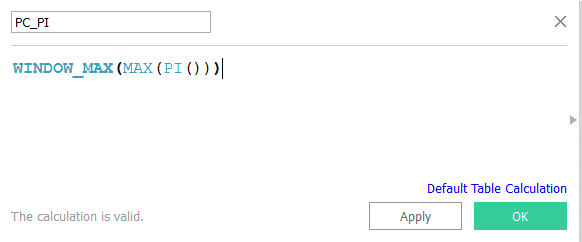4. PC_Depth: This calculation is required to show the remaining part of a circle which is left out from the given value. Calculation is- (1-[PC_value])*180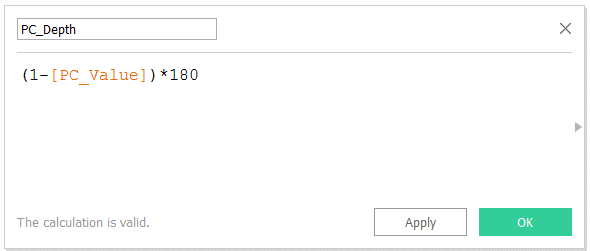5. PC_X: This is used as the X-axis for the chat. Calculation is- SIN([Index]*[PC_PI]/180)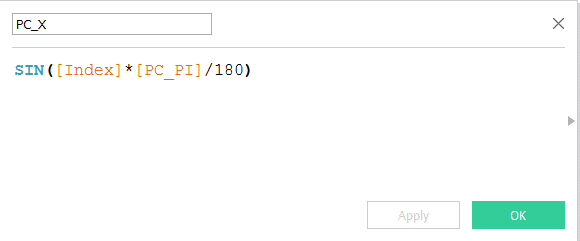6. PC_Y: This is the conditional calculation required for Y-axis. Calculation is-
IF [Index]<[PC_depth] or [Index]> (360-[PC_depth]) THEN
COS([PC_depth]*[PC_PI]/180)
ELSE
COS([Index]*[PC_PI]/180)
END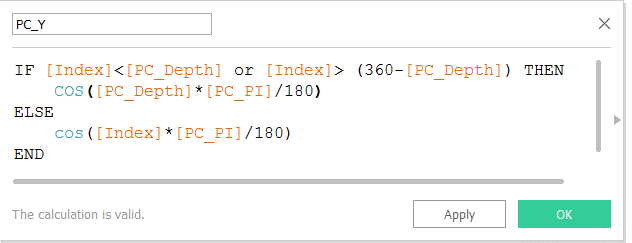7. PC_Y2: This calculation is used for dual Y-axis. Calculation is- COS([Index]*[PC_PI]/180)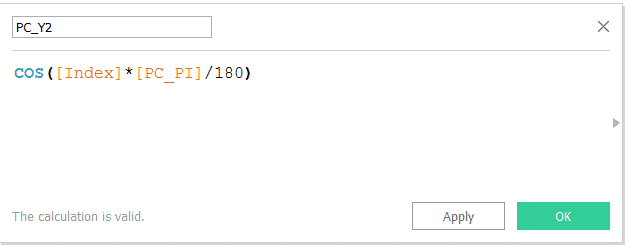8. PC_Color: This calculation is used to define the color of each KPI. Calculation is: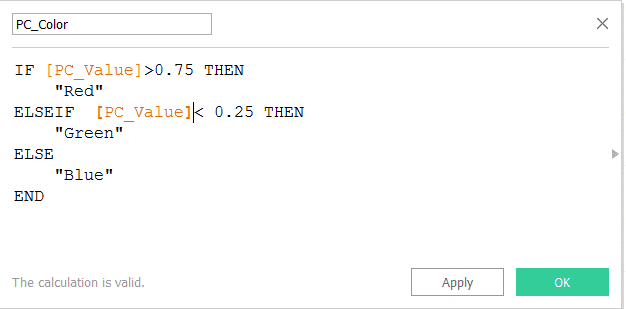5. Now, drag the “KPI”, “PC_Value” and “PC_X” fields to “Columns” shelf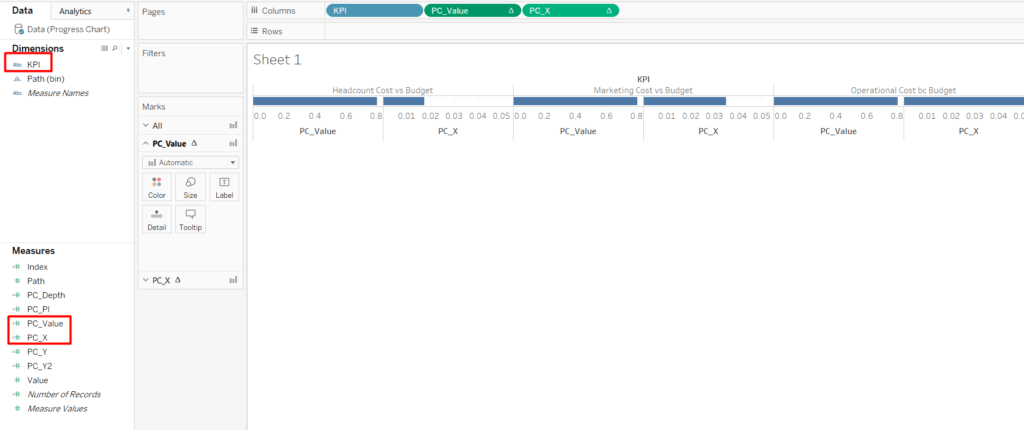6. Convert “PC_Value” field to “Discrete”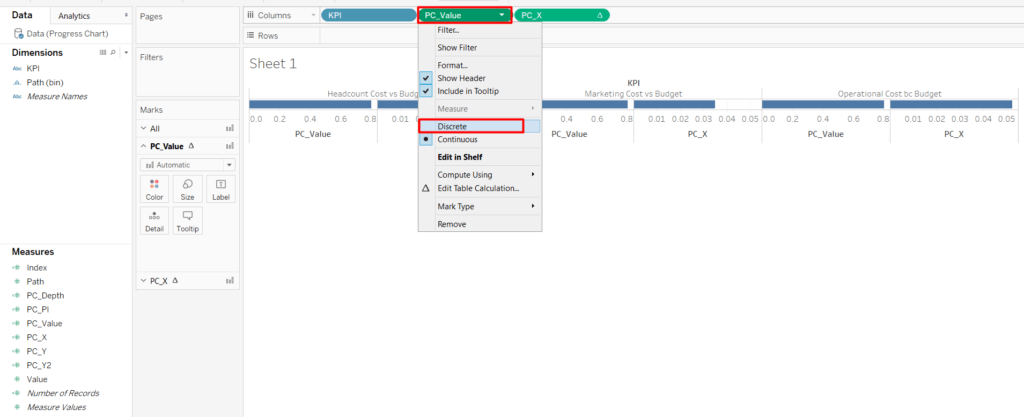7. Drag “PC_Y” field to “Rows” shelf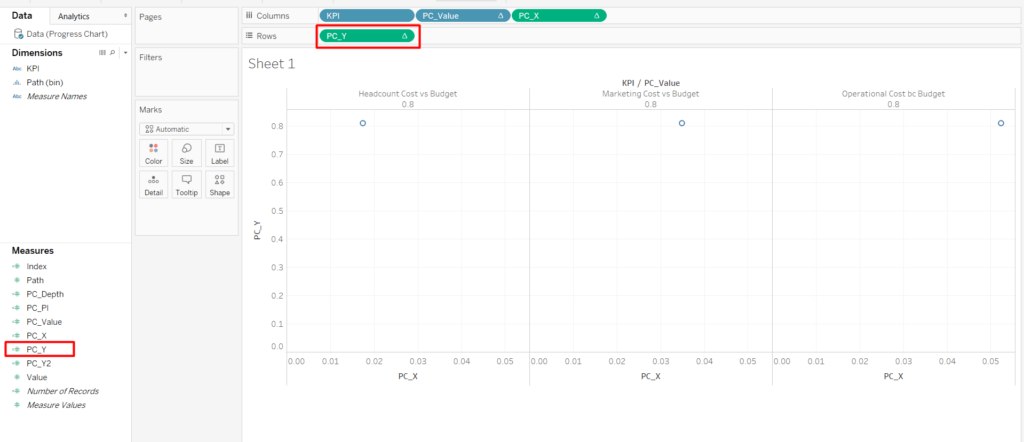8. In the “Marks” card, change the type of chart from “Automatic” to “Polygon”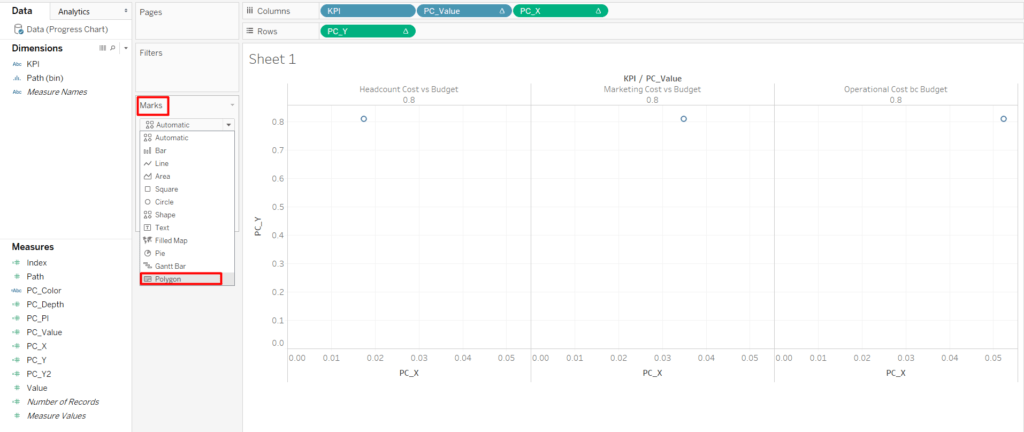9. Now, drag “Path (Bin)” to “Path” and “Detail” ‘marks card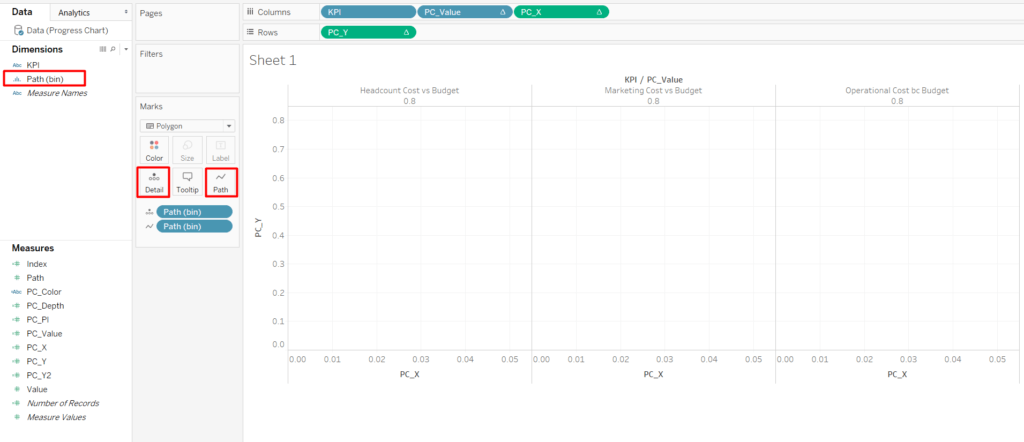10. Change all the Table Calculations as compute using “Path (Bin)”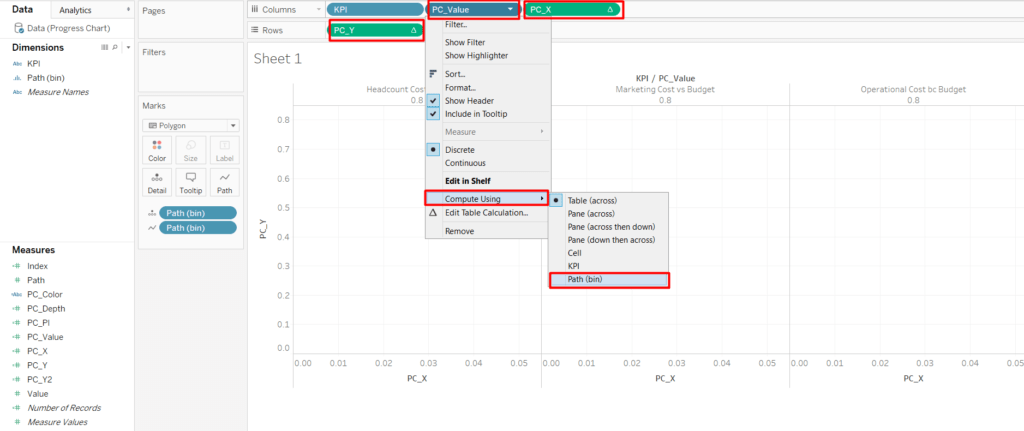11. Once, all the “Table Calculation” of all fields updated to “Path (Bin)”, the chart will look like this: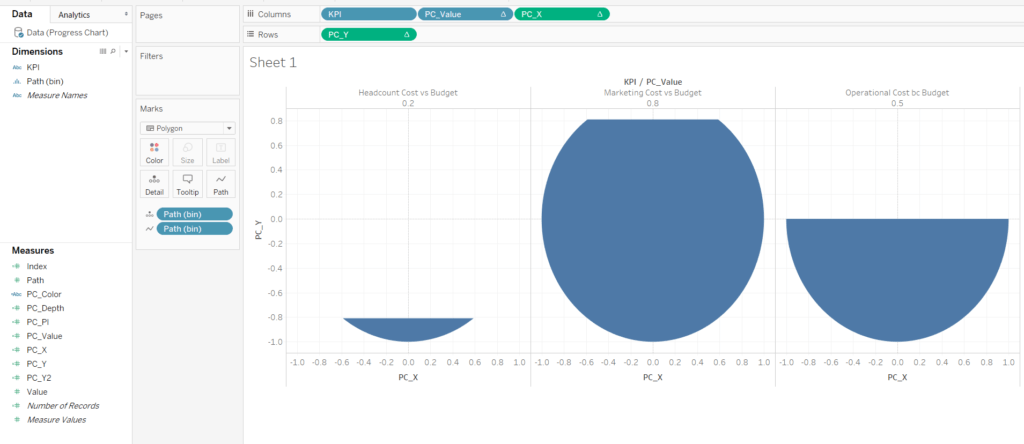12. Drag “PC_Color” field to “Color” marks card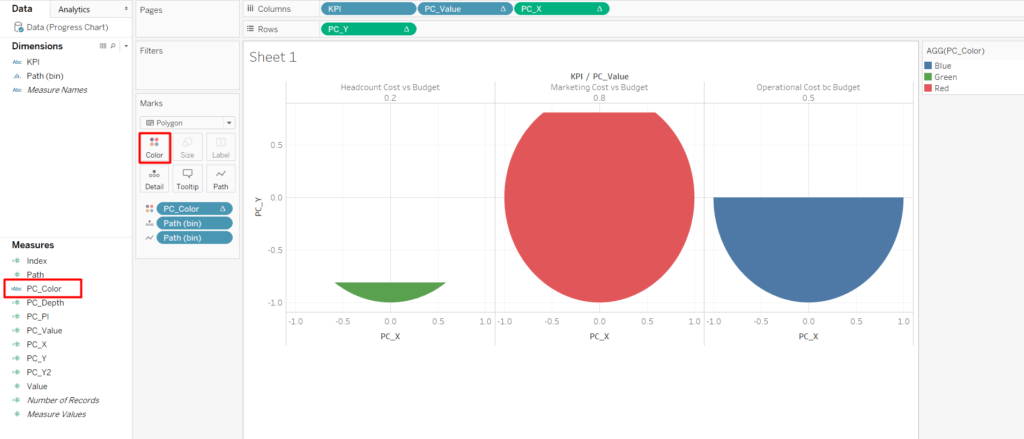13. Now, drag “PC_Y2” and put it next to “PC_Y” in “Rows” shelf, to make the secondary axis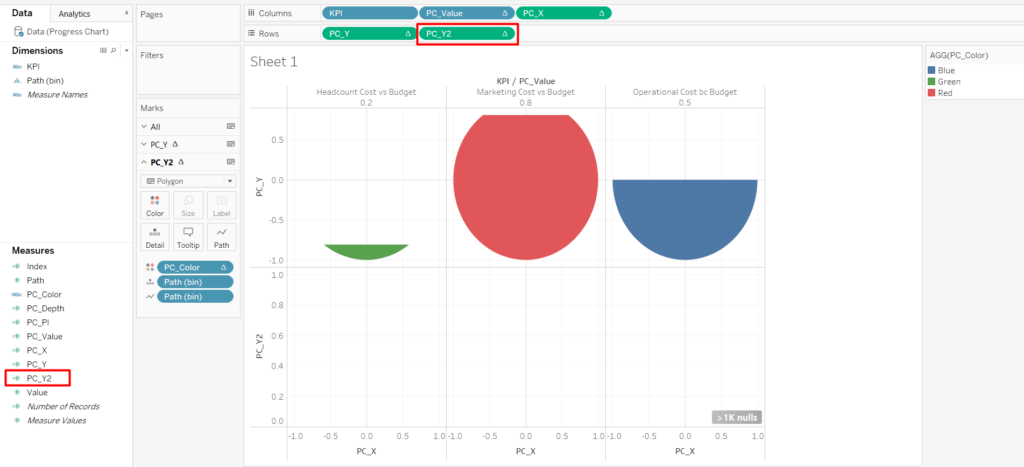14. Select “PC_Y2” and again change the “Table Calculation” as compute using “Path (Bin)”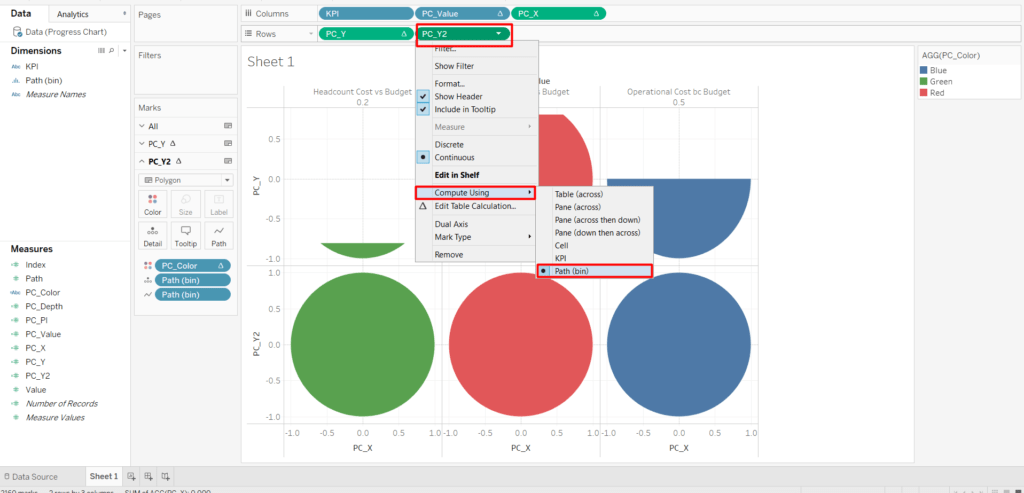15. Remove the “PC_Color” field from “Color” Marks card of “PC_Y2”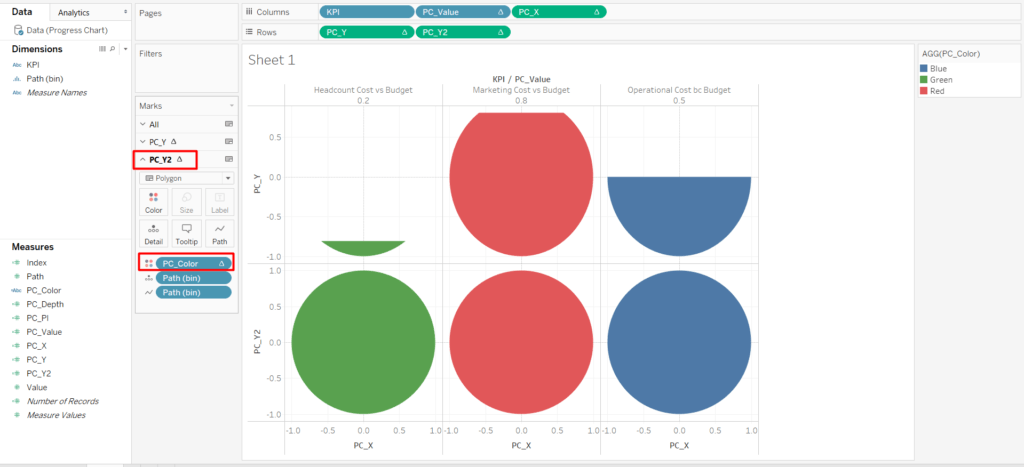16. Remove the “PC_Color” field from “Color” Marks card of “PC_Y2”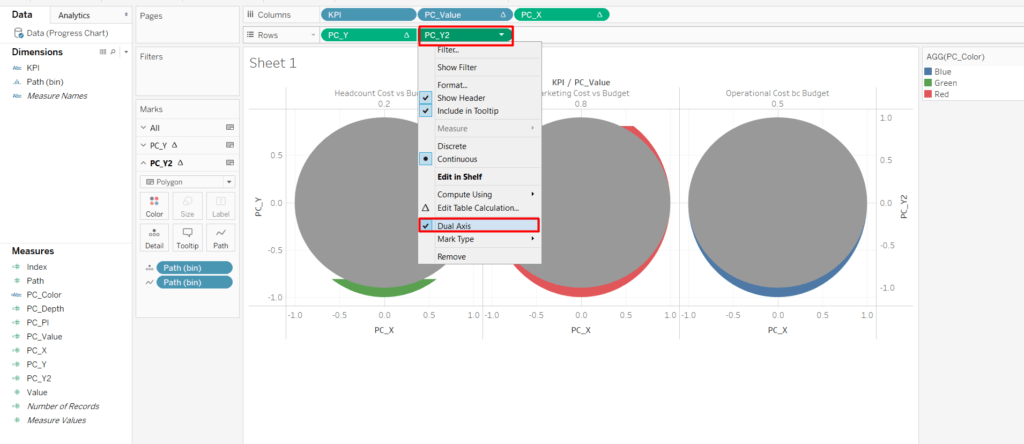17. Select the “Synchronize Axis” option in the secondary “PC_Y2” axis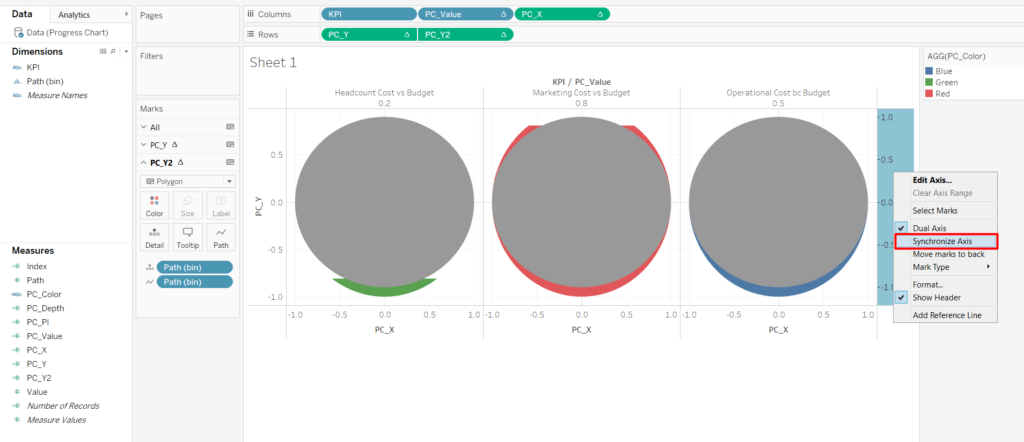18. Next step is to swap the position of “PC_Y” and “PC_Y2” in the rows shelf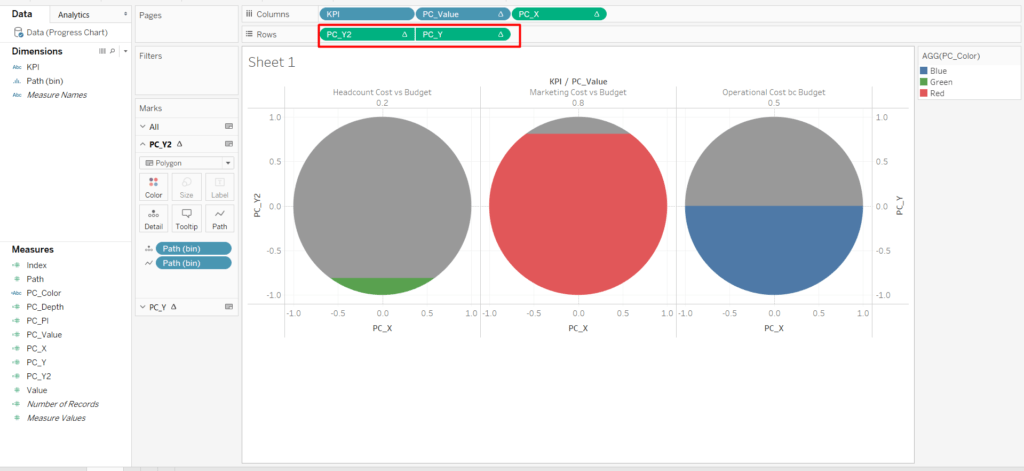19. The last step is to hide the axis headers to make the chart visually more appealing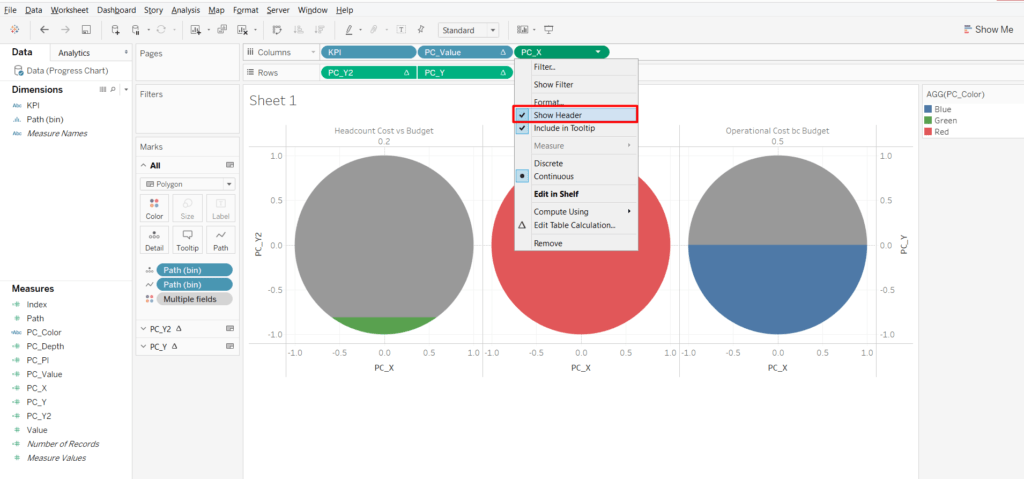20. This is how the “Progress Chart” looks like after the formatting changes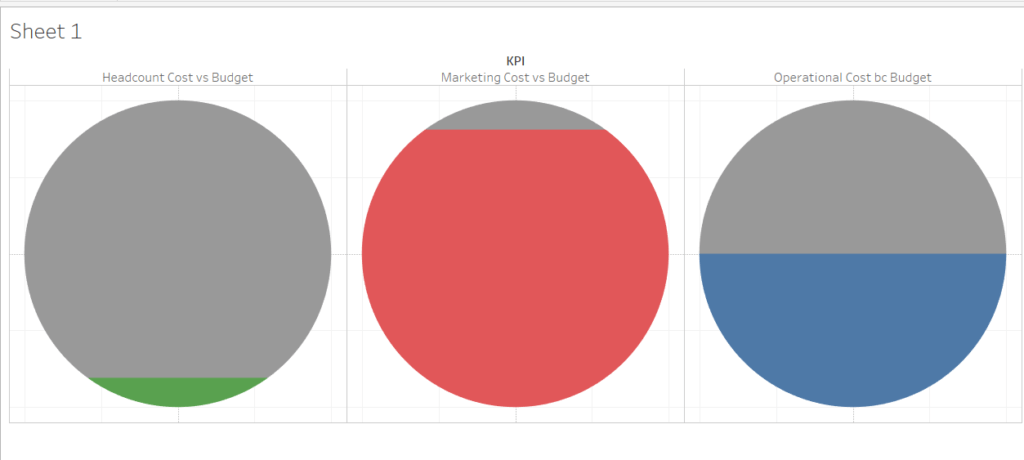• in
• |
• September 24, 2018#### Author1

Subscribe
Notify of